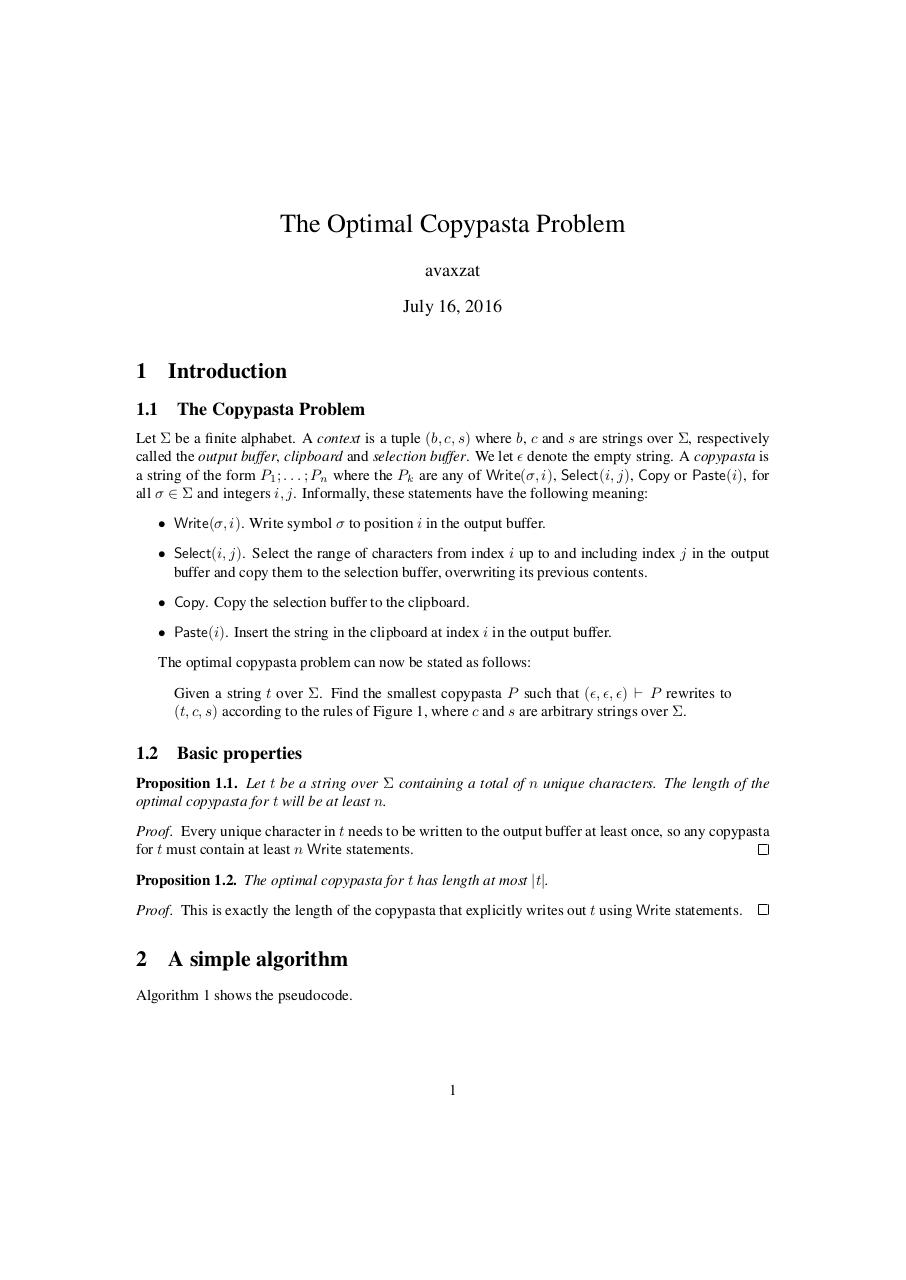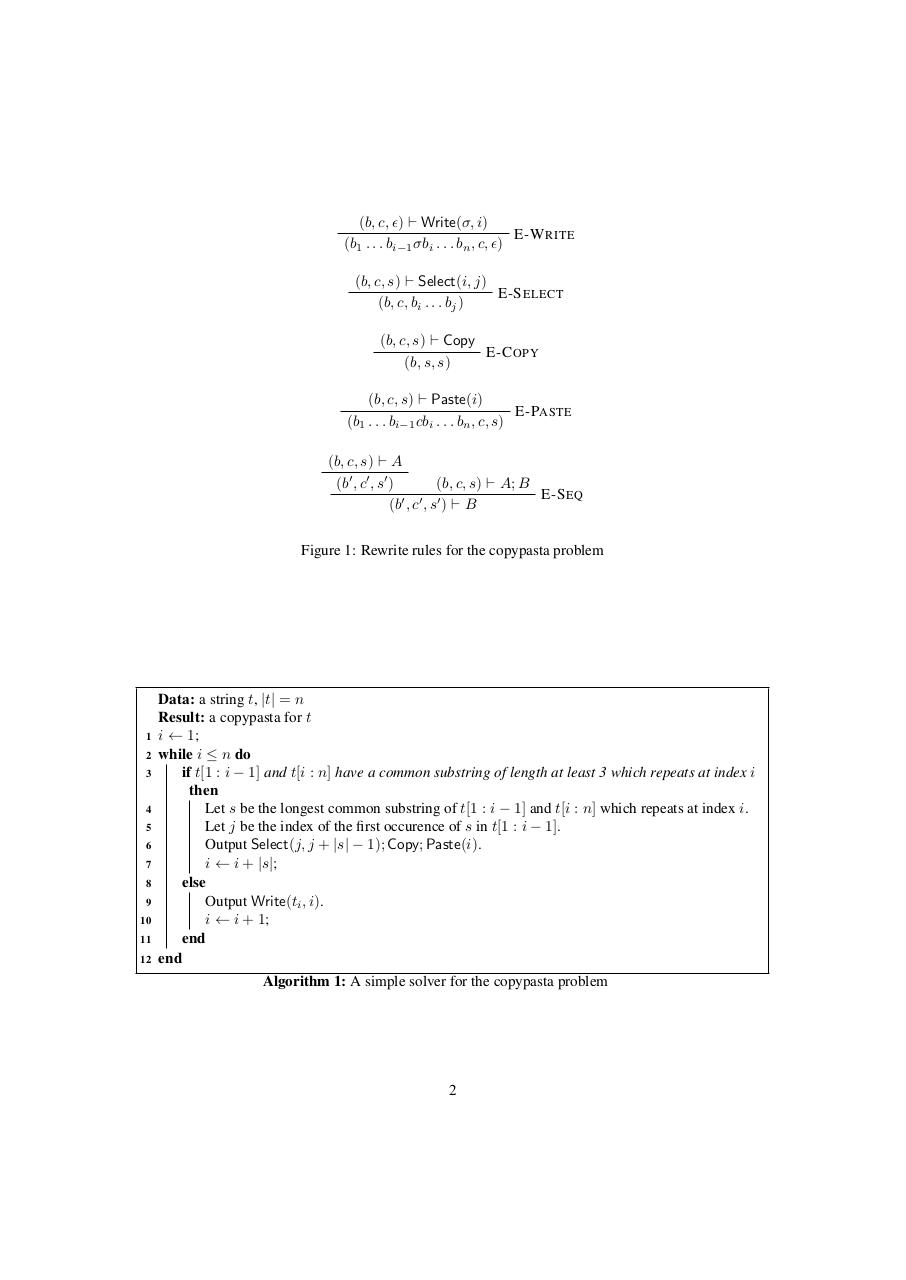# Copypasta .pdf

### File information

Original filename: Copypasta.pdf

This PDF 1.5 document has been generated by TeX / pdfTeX-1.40.16, and has been sent on pdf-archive.com on 16/07/2016 at 16:29, from IP address 81.241.x.x. The current document download page has been viewed 556 times.
File size: 101 KB (2 pages).
Privacy: public file

Copypasta.pdf (PDF, 101 KB)

### Document preview

The Optimal Copypasta Problem
avaxzat
July 16, 2016

1

Introduction

1.1

The Copypasta Problem

Let Σ be a finite alphabet. A context is a tuple (b, c, s) where b, c and s are strings over Σ, respectively
called the output buffer, clipboard and selection buffer. We let  denote the empty string. A copypasta is
a string of the form P1 ; . . . ; Pn where the Pk are any of Write(σ, i), Select(i, j), Copy or Paste(i), for
all σ ∈ Σ and integers i, j. Informally, these statements have the following meaning:
• Write(σ, i). Write symbol σ to position i in the output buffer.
• Select(i, j). Select the range of characters from index i up to and including index j in the output
buffer and copy them to the selection buffer, overwriting its previous contents.
• Copy. Copy the selection buffer to the clipboard.
• Paste(i). Insert the string in the clipboard at index i in the output buffer.
The optimal copypasta problem can now be stated as follows:
Given a string t over Σ. Find the smallest copypasta P such that (, , ) ` P rewrites to
(t, c, s) according to the rules of Figure 1, where c and s are arbitrary strings over Σ.

1.2

Basic properties

Proposition 1.1. Let t be a string over Σ containing a total of n unique characters. The length of the
optimal copypasta for t will be at least n.
Proof. Every unique character in t needs to be written to the output buffer at least once, so any copypasta
for t must contain at least n Write statements.
Proposition 1.2. The optimal copypasta for t has length at most |t|.
Proof. This is exactly the length of the copypasta that explicitly writes out t using Write statements.

2

A simple algorithm

Algorithm 1 shows the pseudocode.

1

(b, c, ) ` Write(σ, i)
E-W RITE
(b1 . . . bi−1 σbi . . . bn , c, )
(b, c, s) ` Select(i, j)
E-S ELECT
(b, c, bi . . . bj )
(b, c, s) ` Copy
E-C OPY
(b, s, s)
(b, c, s) ` Paste(i)
E-PASTE
(b1 . . . bi−1 cbi . . . bn , c, s)
(b, c, s) ` A
(b0 , c0 , s0 )
(b, c, s) ` A; B
E-S EQ
(b0 , c0 , s0 ) ` B
Figure 1: Rewrite rules for the copypasta problem

1
2
3

4
5
6
7
8
9
10
11
12

Data: a string t, |t| = n
Result: a copypasta for t
i ← 1;
while i ≤ n do
if t[1 : i − 1] and t[i : n] have a common substring of length at least 3 which repeats at index i
then
Let s be the longest common substring of t[1 : i − 1] and t[i : n] which repeats at index i.
Let j be the index of the first occurence of s in t[1 : i − 1].
Output Select(j, j + |s| − 1); Copy; Paste(i).
i ← i + |s|;
else
Output Write(ti , i).
i ← i + 1;
end
end
Algorithm 1: A simple solver for the copypasta problem

2#### HTML Code

Copy the following HTML code to share your document on a Website or Blog

#### QR Code### Related keywords